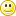FAQ/Collinearity - CBU statistics Wiki
location: FAQ / Collinearity

Collinearity

Its origins, effects, signs, symptoms and cures

Origins: What is Collinearity?

• Collinearity occurs when a predictor is too highly correlated with one or more of the other predictors.

• Sometimes this is referred to as multicollinearity.

• In effect, one or more of the predictors can be closely modelled as a linear combination of the other predictors.

The Impact of Collinearity

• The regression coefficients are very sensitive to minor changes in the data.
• The regression coefficients have large standard errors, which lead to low power for the predictors.
• In the extreme case singularity occurs. The matrix of variances and covariances is singular and cannot be inverted, and and so the regression equation cannot be calculated.

Indices of Collinearity

There are a profusion of numerical measures related to collinearity: Tolerances, Variance Inflation Factors, Condition Indexes and Variance Proportions

Tolerance = 1-R(X)2

= 1-(correlation between predictor X and all the other predictors)2

Tolerance tells us: The amount of overlap between the predictor and all other remaining predictors. The degree of instability in the regression coefficients. Tolerance values less than 0.10 are often considered to be an indication of collinearity.

The VIF tells us: The degree to which the standard error of the predictor is increased due to the predictor’s correlation with the other predictors in the model. VIF values greater than 10 (or, Tolerance values less than 0.10) corresponding to a multiple correlation of 0.95 indicates a multicollinearity may be a problem (Hair Jr, JF, Anderson, RE, Tatham, RL and Black, WC, 1998). Fox and Weisberg also comment that the straightforward VIF can’t be used if there are variables with more than one degree of freedom (e.g. polynomial and other contrasts relating to categorical variables with more than two levels) and recommend using the gvif function (generalized variance inflation factor) in the car package in R in these cases. gvif is the square root of the VIF for individual predictors and thus can be used equivalently. More generally generalized variance-inflation factors consist of the VIF corrected by the number of degrees of freedom (df) of the predictor variable: GVIF = VIF[1/(2*df)] and may be compared to thresholds of 10[1/(2*df)] to assess collinearity using the stepVIF function in R ( see here).

Condition Indices

The standard measure of ill-conditioning in a matrix is the condition index. It will indicate that the inversion of the matrix is numerically unstable with finite-precision numbers ( standard computer floats and doubles ). This indicates the potential sensitivity of the computed inverse to small changes in the original matrix. The Condition Number is computed by finding the square root of (the maximum eigenvalue divided by the minimum eigenvalue). A collinearity problem is indicated (Hair et al, 1998, page 220) when a condition index above the threshold value of 30 accounts for a substantial proportion of variance (0.90 or above) for two or more variables (excluding the constant term).

Correlation Matrix

Construction of a correlation matrix among the explanatory variables will yield indications as to the likelihood that any given couplet of right-hand-side variables are creating multicollinearity problems. Correlation values (off-diagonal elements) of at least .9 are sometimes interpreted as indicating a multicollinearity problem. (Hair et al., 1998).

When to take action

Taken together, they provide information about… whether collinearity is a concern if collinearity is a concern, which predictors are “too” highly correlated Belsley (1991, p. 56) ‘Weak Dependencies’ have condition indices around 5-10 and two or more variance proportions greater than 0.50. ‘Strong Dependencies’ have condition indices around 30 or higher and two or more variance proportions greater than 0.50.

What to do

Convert all the predictors to Z-scores to minimize the effects of rounding errors. (This may not be sufficient.) Delete some of the predictors that are too highly correlated, but this may lead to model misspecification! Collect additional data…in the hope that additional data will reduce the collinearity. Use principal components or factor analysis to consolidate the information contained in your predictors. Use ridge regression or robust regression methods.

Belsley, David Alan (1991) Conditioning diagnostics : collinearity and weak data in regression. New York ; Chichester : Wiley. [Library details UL: 202.c.99.50 South Wing, Floor 5]

Belsley, David Alan (1980). Regression diagnostics : identifying influential data and sources of collinearity. New York ; Chichester : Wiley. [Library details B&GM: QA278.2 .B44 1980; UL: 202.c.98.17 South Wing, Floor 5]

Fox J and Weisberg S (2011) An R companion to applied regression. Second Edition. Sage:Thousand Oaks.

Hair Jr., JF, Tatham, RL, Anderson, RE and Black, W (1998, 2004) Multivariate Data Analysis (5th edition). Prentice-Hall:Englewood Cliffs, NJ. This accessible and comprehensive text features plenty of illustrations and rules of thumb.There are also sixth (2005)and seventh (2009) editions by Hair Jr, JF, Black, B, Babin, B, Anderson, RE, Tatham, RL published by Pearson International.

What to do if you encounter collinearity

[Last updated on 27 November, 2003]

These pages are maintained by Ian Nimmo-Smith and Peter Watson